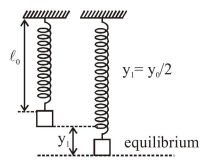# When a particle of mass

Question:

When a particle of mass $m$ is attached to a vertical spring of spring constant $\mathrm{k}$ and released, its motion is described by $y(t)=y_{0} \sin ^{2} \omega t$, where ' $y$ ' is measured from the lower end of unstretched spring. Then $\omega$ is :

1. $\sqrt{\frac{\mathrm{g}}{\mathrm{y}_{0}}}$

2. $\sqrt{\frac{g}{2 y_{0}}}$

3. $\frac{1}{2} \sqrt{\frac{g}{y_{0}}}$

4. $\sqrt{\frac{2 g}{y_{0}}}$

Correct Option: , 2

Solution:

$\mathrm{y}=\mathrm{y}_{0} \sin ^{2} \omega \mathrm{t}$

$y=\frac{y_{0}}{2}(1-\cos 2 \omega t)$

$y-\frac{y_{0}}{2}=-\frac{y_{0}}{2} \cos 2 \omega t$

Amplitude : $\frac{\mathrm{y}_{0}}{2}$$\frac{y_{0}}{2}=\frac{m g}{K}$

$2 \omega=\sqrt{\frac{K}{m}}=\sqrt{\frac{2 g}{y_{0}}}$

$\omega=\sqrt{\frac{g}{2 y_{0}}}$

Ans.(2)